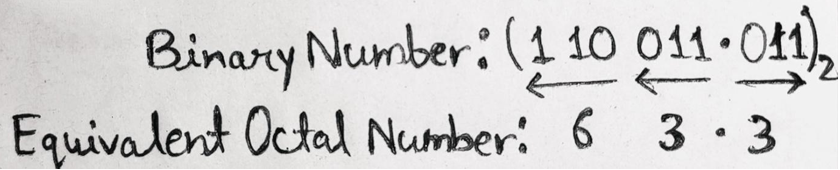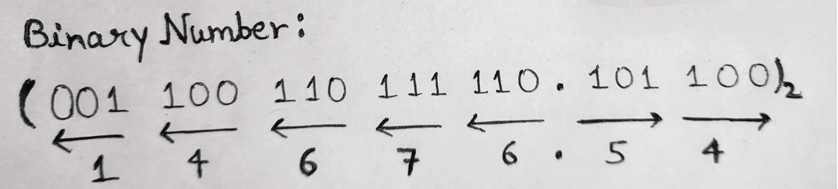# Binary to Octal Number System Conversion

In this tutorial, we will learn about the conversion of binary to octal number systems with the help of examples. By Saurabh Gupta Last updated : May 10, 2023

Prerequisite: Number systems

## Binary to Octal Number System Conversion

To convert binary numbers into octal numbers, we first have to understand the relationship between binary and octal numbers.

Octal Number Binary Number
0000
1001
2010
3011
4100
5101
6110
7111

In octal number system, we have eight digits ranging from 0 to 7 which can be represented using three-bit binary numbers in 23 = 8 ways, so starting from the least significant bit of the binary number, we group three successive bits of the binary number to get its equivalent octal number as seen from the table above.

In an integral part, the grouping of three bits is done from the right side to the left side whereas in the fractional part the grouping of three bits is done from left to right and then convert it to its equivalent octal symbol.

In the process of grouping three bits, one or two bits can be added to the left of the MSB in an integral part and/or to the right of the LSB bit of the fractional part of the binary number.

### Example 1: Convert (110011.011)2 to ( ? )8Therefore, (110011.011)2 = (63.3)8

### Example 2: Convert (110011011110.1011)2 to ( ? )8Therefore, (110011011110.1011)2 = (14676.54)8

Note: In the Example 2, to make a group of three bits, we have added two additional bits to the left of MSB in an integral part and two additional bits to the right of LSB in the fractional part.